# Linear Models for Predicting Continuous Values¶

## Linear Structure¶

$\newcommand{\xv}{\mathbf{x}} \newcommand{\Xv}{\mathbf{X}} \newcommand{\yv}{\mathbf{y}} \newcommand{\zv}{\mathbf{z}} \newcommand{\av}{\mathbf{a}} \newcommand{\Wv}{\mathbf{W}} \newcommand{\wv}{\mathbf{w}} \newcommand{\tv}{\mathbf{t}} \newcommand{\Tv}{\mathbf{T}} \newcommand{\muv}{\boldsymbol{\mu}} \newcommand{\sigmav}{\boldsymbol{\sigma}} \newcommand{\phiv}{\boldsymbol{\phi}} \newcommand{\Phiv}{\boldsymbol{\Phi}} \newcommand{\Sigmav}{\boldsymbol{\Sigma}} \newcommand{\Lambdav}{\boldsymbol{\Lambda}} \newcommand{\half}{\frac{1}{2}} \newcommand{\argmax}{\underset{#1}{\operatorname{argmax}}} \newcommand{\argmin}{\underset{#1}{\operatorname{argmin}}}$

Given $N$ observations, $\xv_n$, for $n=1,\ldots,N$, and target values, $t_n$, for $n=1,\ldots,N$, what is the simplest model, $g(\xv)$, you can think of?

$$g(\xv) = 0$$

or maybe

$$g(\xv) = c$$

What is next simplest model?

\begin{align*} g(\xv;\wv) &= w_0 + w_1 x_1 + w_2 x_2 + \cdots + w_D x_D \\ &= w_0 + \sum_{i=1}^D w_i x_i \\ & = \sum_{i=0}^D w_i x_i \mbox{, where } x_0 = 1 \\ &= \wv^T \xv \end{align*}
• This is nice because it is linear in the parameters $\wv$; optimizations based on derivatives might be solvable analytically.
• This is not so nice, because it is also linear in the inputs, $\xv$; greatly limits the complexity of the model.
• But, a model linear in the inputs might be the best you can do for many cases, such as a sparsely sampled distribution, process, population, thing...whatever it is you want to model.

## Fitting Data Samples with a Linear Model¶Force exerted by spring is proportional to its length. The potential energy stored in the spring is proportional to the square of its length. Say we want the rod to settle at position that minimizes the potential energy in the springs. \begin{align*} \sum_{n=1}^N (t_n - g(\xv_n;\wv))^2 \end{align*}

If $g$ is an affine (linear + constant) function of $x$, $$g(\xv;\wv) = w_0 + w_1 x$$ with parameters $\wv = (w_0, w_1)$, which parameter values give best fit? $$\wv_{\mbox{best}} = \argmin{\wv} \sum_{n=1}^N (t_n - g(x_n ; \wv))^2$$

Set derivative (gradient) with respect to $\wv$ to zero and solve for $\wv$. Let's do this with matrices.

The matrix formulas are a bit simpler if we assume that $w_0$ is multipled by the constant 1, and that $x_{i, 0}$, the first component of sample $i$, is the constant 1.

Collect all targets into matrix $T$ and $x$ samples into matrix $X$. ($N$=number samples, $D$=sample dimensionality) \begin{align*} T &= \begin{bmatrix} t_1 \\ t_2 \\ \vdots \\ t_N \end{bmatrix} \\ X &= \begin{bmatrix} x_{1,0} & x_{1,1} & x_{1,2} & \dotsc & x_{1,D} \\ x_{2,0} & x_{2,1} & x_{2,2} & \dotsc & x_{2,D} \\ \vdots \\ x_{N,0} & x_{N,1} & x_{N,2} & \dotsc & x_{N,D} \end{bmatrix}\\ \wv &= \begin{bmatrix} w_0 \\ w_1 \\ \vdots \\ w_D \end{bmatrix} \end{align*}

Collection of all differences is $T - X\wv$, which is an $N \times 1$ matrix. To form the square of all values and add them up, just do a dot product $(T-X\wv)^T (T-X\wv)$. This only works if the value we are predicting is a scalar, which means $T$ is a column matrix. If we want to predict more than one value for each sample, $T$ will have more than one column. Let's continue with the derivation assuming $T$ has $K$ columns, meaning we want a linear model with $K$ outputs.

To find the best value for $\wv$, we take the derivative of the sum of squared error objective, set it equal to 0 and solve for $\wv$.

\begin{align*} \frac{\partial \sum_{n=1}^N (\tv_n - g(\xv_n;\wv))^2}{\partial \wv} &= -2 \sum_{n=1}^N (\tv_n - g(\xv_n;\wv) \frac{\partial g(\xv_n;\wv)}{\partial \wv}\\ &= -2 \sum_{n=1}^N (\tv_n - \xv_n^T \wv) \frac{\partial \xv_n^T \wv}{\partial \wv}\\ &= -2 \sum_{n=1}^N (\tv_n - \xv_n^T \wv) \xv_n^T \end{align*}

Here's where we get the benefit of expressing the $\xv_n$ and $t_n$ samples as matrices. The sum can be performed with a dot product:

\begin{align*} \frac{\partial \sum_{n=1}^N (\tv_n - g(\xv_n;\wv))^2}{\partial \wv} &= -2 \sum_{n=1}^N (\tv_n - \xv_n^T \wv) \xv_n^T\\ &= -2 \Xv^T (\Tv - \Xv \wv) \end{align*}

Check the sizes and shapes of each matrix in the last equation above.

Now we can set this equal to zero and solve for $\wv$.

\begin{align*} -2 \Xv^T (\Tv - \Xv \wv) &= 0\\ \Xv^T (\Tv - \Xv \wv) &= 0\\ \Xv^T \Tv &= \Xv^T \Xv \wv\\ \wv &= (\Xv^T \Xv)^{-1} \Xv^T \Tv \end{align*}

And, in python

w = np.linalg.inv(X.T @ X), X.T @ T)



or, you may use the solve function that assumes $\Xv^T \Xv$ is full rank (no linearly dependent columns),

w = np.linalg.solve(X.T @ X, X.T @ T)



or, better yet, use the lstsq function that does not make that assumption.

w = np.linalg.lstsq(X.T @ X, X.T @ T))



The lstsq and solve functions can be written with simpler arguments, like

w = np.linalg.lstsq(X, T))



because they are designed to find the value of $\wv$ that minimized the squared error between $X \wv$ and $T$. Using the matrix products as arguments will simplify our implementation of ridge regression a little bit later in this course. For now, let's use the simpler version.

## Incremental Way¶

But what if you have thousands or millions of samples? $X$ and $T$ can be quite large. To avoid dealing with matrix operations on huge matrices, we can derive a sequential algorithm for finding $\wv$ by using the fact that a derivative of a sum is the sum of the derivatives. We will now express this derivative as a gradient, which is a vector or matrix of derivatives.

\begin{align*} g(\xv_n, \wv) &= w_0 + w_1 x_{n,1} + w_2 x_{n,2} + \cdots + w_D x_{n,D} = \xv_n^T \wv\\ E(\Xv, \Tv, \wv) &= \sum_{n=1}^N (t_n - g(\xv_n, \wv))^2\\ \nabla_\wv E(\Xv, \Tv, \wv) &= \nabla_\wv \left ( \sum_{n=1}^N (t_n - g(\xv_n, \wv))^2 \right )\\ &= \sum_{n=1}^N \nabla_\wv (t_n - g(\xv_n, \wv))^2\\ &= \sum_{n=1}^N 2 (t_n - g(\xv_n, \wv)) \nabla_\wv (t_n - g(\xv_n, \wv)) \\ &= \sum_{n=1}^N 2 (t_n - g(\xv_n, \wv)) (-1) \nabla_\wv g(\xv_n, \wv) \\ &= \sum_{n=1}^N 2 (t_n - g(\xv_n, \wv)) (-1) \nabla_\wv (\xv_n^T \wv) \\ &= \sum_{n=1}^N 2 (t_n - g(\xv_n, \wv)) (-1) \xv_n \\ &= -2 \sum_{n=1}^N (t_n - g(\xv_n, \wv)) \xv_n \\ \end{align*}

Instead of summing over all $N$ samples, what if we just update $\wv$ after each sample based on the gradient of $E$ for that sample? The gradient for a sample $n$ can be considered as a limited, or noisy, sample of the true gradient. Thus, we can take a small step in the direction of the negative gradient to try to bring a current guess at the weight vector, $\wv^{(k)}$, on iteration $k$ to a new value, $\wv^{(k+1)}$, on iteration $k+1$ that is closer to a value that reduces the overall error. This kind of update is called "stochastic approximation".

\begin{align*} \wv^{(k+1)} &= \wv^{(k)} - (-2) \rho (t_n - g(\xv_n, \wv)) \xv_n\\ &= \wv^{(k)} + \rho (t_n - g(\xv_n, \wv)) \xv_n \end{align*}

For this sequential algorithm to converge, $\rho$ must decrease with each iteration, not too fast but not too slow.

This algorithm is called the least mean squares (LMS) algorithm developed by Widrow and Hoff. It is now often referred to as the ''stochastic gradient descent'' algorithm, or SGD.

If we have two output variables, like mpg and horsepower, then $t_n$ is no longer a scalar. How do we deal with that? Well, to predict two variables, we need two linear models. We can do this by changing $\wv$ from a single column matrix to a two-column matrix. The first column could contain the weights used to predict mpg, and the second column could contain weights to predict horsepower. Now our linear model is

$$g(\xv_n, \wv) = \xv_n^T \wv$$

Humm, no change here! This is the beauty of using matrix math. The input vector $\xv_n$ is dotted with each of the two columns of $\wv$, resulting in two values, or a two-component resulting vector, giving the predictions for mpg and horsepower.

What changes do we need to make to the SGD update formula? What else must we modify, other than $\wv$? For each sample, $n$, we must specify two target values, for mpg and horsepower. So $t_n$ is no longer a scalar, but now has two values in a vector, or $\tv_n$. To update the weights $\wv$ we must multiply each error by each input component. This does sound like a double loop. Well, in the last equation above we already used matrix math and numpy broadcasting once in

\begin{align*} \wv^{(k+1)} &= \wv^{(k)} + \rho \; (t_n - g(\xv_n, \wv)) \; \xv_n \end{align*}

to remove the loop over all of the components in $\wv_n$ and $\xv_n$. Now we will use broadcasting again to remove a loop over target components, in $\tv_n$. We must take care to make sure the matrices are of the right shape in the matrix operations, and that the resulting matrix is the correct shape for $\wv$. Here we follow the convention that vectors are column vectors.

\begin{align*} \wv^{(k+1)} &= \wv^{(k)} + \rho \; \xv_n \; (\tv_n^T - g(\xv_n, \wv))) \end{align*}

Let's see, $\rho$ is a scalar, $\xv_n$ is $D+1\times 1$, a column vector with $D+1$ components (counting the constant 1), $\tv_n$ is $K\times 1$ if we have $K$ outputs, so $\tv_n^T$ is $1\times K$ and $g(\xv_n, \wv) = \xv_n^T \wv$ is also $1\times K$. Stringing these dimensions together in the calculation gives us $(D+1\times 1) (1\times K)$ which results in $D+1\times K$ exactly the correct shape for our weight matrix $\wv$!

In Python, the update to the weight matrix for the $n^{th}$ sample is just

 w += rho * X1[n:n+1, :].T * (T[n:n+1, :] - predicted)



The long, boring, non-matrix way to update each element of w would look like

 nOutputs = T.shape
nInputs = X1.shape
for k in range(nOutputs):
for i in range(nInputs):
w[i,k] += rho * X1[n:n+1, i] * (T[n:n+1, k] - predicted[k])



So many lines of code can lead to more bugs!!

## Example of SGD in Action¶

In :
import numpy as np
import matplotlib.pyplot as plt
from IPython.display import display, clear_output  # for the following animation


Let's make some silly data to play with. Make 100 samples of random $x$ values between 0 and 10, and assign the target for each sample to be $2 - 0.1 X + (X - 6)^2 + \epsilon$, where $\epsilon$ is a bit of noise as a random value from a Normal distribution with mean 0 and standard deviation 0.1.

In :
n_samples = 100
X = np.random.uniform(0, 10, (n_samples, 1))
T = 2 - 0.1 * X + 0.05 * (X - 6)**2 + np.random.normal(0, 0.1, (n_samples,1))

In :
np.random.normal?

In :
plt.plot(X, T)

Out:
[<matplotlib.lines.Line2D at 0x7febec96d0d0>]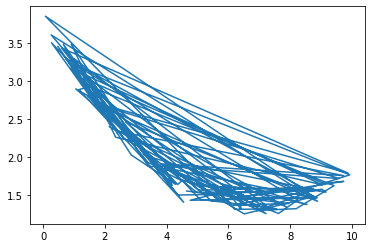In :
plt.plot(X, T, 'o')

Out:
[<matplotlib.lines.Line2D at 0x7febec8c7cd0>]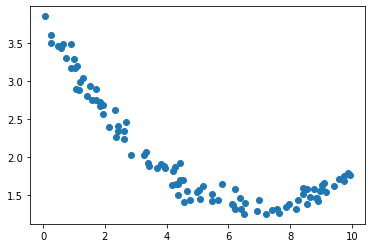Do you think we can fit a linear model to this data?

First, let's modify the $X$ input matrix to include an initial column of constant 1.

In :
X1 = np.insert(X, 0, 1, axis=1)

X1.shape, T.shape

Out:
((100, 2), (100, 1))
In :
learning_rate = 0.01
n_samples = X1.shape  # number of rows in data equals the number of samples

w = np.zeros((2, 1))                # initialize the weights to zeros
for epoch in range(10):             # train for this many epochs, or passes through the data set
for n in range(n_samples):
y = X1[n:n + 1, :] @ w      # predicted value, y, for sample n
error = T[n:n + 1, :] - y
# update weights by fraction of negative derivative of square error with respect to weights
w += learning_rate * X1[n:n + 1, :].T * error

print(w)

[[ 2.78586497]
[-0.11444579]]


Let's see how well this linear model (defined by these resulting weights) fits the data. To do so, we can plot the model's predictions on top of the plot of actual data.

In :
plt.plot(X, T, 'o', label='Data')
plt.plot(X, X1 @ w, 'ro', label='Predicted')
plt.legend();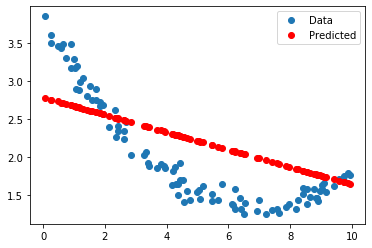Let's animate each step by drawing the predictions made by the linear model as weights are updated.

In :
def run(rho, n_epochs, stepsPerFrame=10):

# Initialize weights to all zeros
# For this demonstration, we will have one variable input. With the constant 1 input, we have 2 weights.
w = np.zeros((2,1))

# Collect the weights after each update in a list for later plotting.
# This is not part of the training algorithm
ws = [w.copy()]

# Create a bunch of x values for plotting
xs = np.linspace(0, 10, 100).reshape((-1,1))
xs1 = np.insert(xs, 0, 1, axis=1)

fig = plt.figure(figsize=(8, 8))

# For each pass (one epoch) through all samples ...
for iter in range(n_epochs):
# For each sample ...
for n in range(n_samples):

# Calculate prediction using current model, w.
#    n:n+1 is used instead of n to preserve the 2-dimensional matrix structure
predicted = X1[n:n+1,:] @ w

# Update w using negative gradient of error for nth sample
w += rho * X1[n:n+1, :].T * (T[n:n+1, :] - predicted)

# Add new w to our list of past w values for plotting
ws.append(w.copy())

if n % stepsPerFrame == 0:
fig.clf()

# Plot the X and T data.
plt.subplot(2, 1, 1)
plt.plot(X, T, 'o', alpha=0.6, label='Data')
plt.plot(X[n,0], T[n], 'ko', ms=10, label='Last Trained Sample')

# Plot the output of our linear model for a range of x values
plt.plot(xs, xs1 @ w, 'r-', linewidth=5, label='Model')
plt.xlabel('$x$')
plt.legend(loc='upper right')
plt.xlim(0, 10)
plt.ylim(0, 5)

# In second panel plot the weights versus the epoch number
plt.subplot(2, 1, 2)
plt.plot(np.array(ws)[:, :, 0])
plt.xlim(0, n_epochs * n_samples)
plt.ylim(-1, 3)
plt.legend(('$w_0$', '$w_1$'))

clear_output(wait=True)
display(fig)

clear_output(wait=True)

return w

In :
run(0.01, n_epochs=1, stepsPerFrame=1)

Out:
array([[0.60852192],
[0.2490183 ]])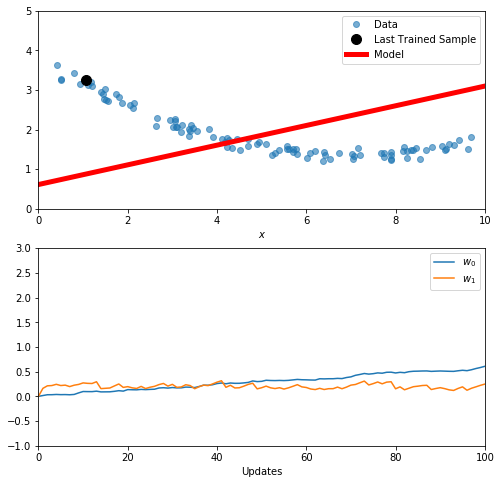In :
run(0.01, n_epochs=20, stepsPerFrame=10)

Out:
array([[ 2.83795596],
[-0.1617015 ]])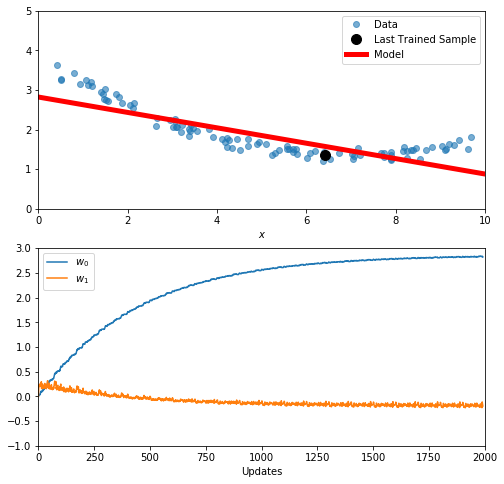## Application¶

Now we can play with some real data.

Try the automobile data set at UCI Machine Learning Database Repository. Download auto-mpg.data and auto-mpg.names from the "Data Folder" link near the top of the web page.

In :
!curl -O http://mlr.cs.umass.edu/ml/machine-learning-databases/auto-mpg/auto-mpg.data
!curl -O http://mlr.cs.umass.edu/ml/machine-learning-databases/auto-mpg/auto-mpg.names

  % Total    % Received % Xferd  Average Speed   Time    Time     Time  Current
100 30286  100 30286    0     0   122k      0 --:--:-- --:--:-- --:--:--  122k
% Total    % Received % Xferd  Average Speed   Time    Time     Time  Current
100  1660  100  1660    0     0  14821      0 --:--:-- --:--:-- --:--:-- 14821


First, take a look at auto-mpg.names. There you will learn that there are 398 samples, each with 8 numerical attributes and one string attribute. Their names are

1. mpg: continuous
2. cylinders: multi-valued discrete
3. displacement: continuous
4. horsepower: continuous
5. weight: continuous
6. acceleration: continuous
7. model year: multi-valued discrete
8. origin: multi-valued discrete
9. car name: string (unique for each instance)

First step, read it into python and look at it.

In :
import pandas  # to help with reading csv file with missing values


Take a look at a few lines of the data file to figure out how to read it in.

In :
!head -40 auto-mpg.data

18.0   8   307.0      130.0      3504.      12.0   70  1	"chevrolet chevelle malibu"
15.0   8   350.0      165.0      3693.      11.5   70  1	"buick skylark 320"
18.0   8   318.0      150.0      3436.      11.0   70  1	"plymouth satellite"
16.0   8   304.0      150.0      3433.      12.0   70  1	"amc rebel sst"
17.0   8   302.0      140.0      3449.      10.5   70  1	"ford torino"
15.0   8   429.0      198.0      4341.      10.0   70  1	"ford galaxie 500"
14.0   8   454.0      220.0      4354.       9.0   70  1	"chevrolet impala"
14.0   8   440.0      215.0      4312.       8.5   70  1	"plymouth fury iii"
14.0   8   455.0      225.0      4425.      10.0   70  1	"pontiac catalina"
15.0   8   390.0      190.0      3850.       8.5   70  1	"amc ambassador dpl"
15.0   8   383.0      170.0      3563.      10.0   70  1	"dodge challenger se"
14.0   8   340.0      160.0      3609.       8.0   70  1	"plymouth 'cuda 340"
15.0   8   400.0      150.0      3761.       9.5   70  1	"chevrolet monte carlo"
14.0   8   455.0      225.0      3086.      10.0   70  1	"buick estate wagon (sw)"
24.0   4   113.0      95.00      2372.      15.0   70  3	"toyota corona mark ii"
22.0   6   198.0      95.00      2833.      15.5   70  1	"plymouth duster"
18.0   6   199.0      97.00      2774.      15.5   70  1	"amc hornet"
21.0   6   200.0      85.00      2587.      16.0   70  1	"ford maverick"
27.0   4   97.00      88.00      2130.      14.5   70  3	"datsun pl510"
26.0   4   97.00      46.00      1835.      20.5   70  2	"volkswagen 1131 deluxe sedan"
25.0   4   110.0      87.00      2672.      17.5   70  2	"peugeot 504"
24.0   4   107.0      90.00      2430.      14.5   70  2	"audi 100 ls"
25.0   4   104.0      95.00      2375.      17.5   70  2	"saab 99e"
26.0   4   121.0      113.0      2234.      12.5   70  2	"bmw 2002"
21.0   6   199.0      90.00      2648.      15.0   70  1	"amc gremlin"
10.0   8   360.0      215.0      4615.      14.0   70  1	"ford f250"
10.0   8   307.0      200.0      4376.      15.0   70  1	"chevy c20"
11.0   8   318.0      210.0      4382.      13.5   70  1	"dodge d200"
9.0    8   304.0      193.0      4732.      18.5   70  1	"hi 1200d"
27.0   4   97.00      88.00      2130.      14.5   71  3	"datsun pl510"
28.0   4   140.0      90.00      2264.      15.5   71  1	"chevrolet vega 2300"
25.0   4   113.0      95.00      2228.      14.0   71  3	"toyota corona"
25.0   4   98.00      ?          2046.      19.0   71  1	"ford pinto"
19.0   6   232.0      100.0      2634.      13.0   71  1	"amc gremlin"
16.0   6   225.0      105.0      3439.      15.5   71  1	"plymouth satellite custom"
17.0   6   250.0      100.0      3329.      15.5   71  1	"chevrolet chevelle malibu"
19.0   6   250.0      88.00      3302.      15.5   71  1	"ford torino 500"
18.0   6   232.0      100.0      3288.      15.5   71  1	"amc matador"
14.0   8   350.0      165.0      4209.      12.0   71  1	"chevrolet impala"
14.0   8   400.0      175.0      4464.      11.5   71  1	"pontiac catalina brougham"

In :
df = pandas.read_csv('auto-mpg.data')
df

Out:
18.0 8 307.0 130.0 3504. 12.0 70 1\t"chevrolet chevelle malibu"
0 15.0 8 350.0 165.0 3693. 11...
1 18.0 8 318.0 150.0 3436. 11...
2 16.0 8 304.0 150.0 3433. 12...
3 17.0 8 302.0 140.0 3449. 10...
4 15.0 8 429.0 198.0 4341. 10...
... ...
392 27.0 4 140.0 86.00 2790. 15...
393 44.0 4 97.00 52.00 2130. 24...
394 32.0 4 135.0 84.00 2295. 11...
395 28.0 4 120.0 79.00 2625. 18...
396 31.0 4 119.0 82.00 2720. 19...

397 rows × 1 columns

In :
pandas.read_csv?


In :
df = pandas.read_csv('auto-mpg.data', header=None, delim_whitespace=True)
df

Out:
0 1 2 3 4 5 6 7 8
0 18.0 8 307.0 130.0 3504.0 12.0 70 1 chevrolet chevelle malibu
1 15.0 8 350.0 165.0 3693.0 11.5 70 1 buick skylark 320
2 18.0 8 318.0 150.0 3436.0 11.0 70 1 plymouth satellite
3 16.0 8 304.0 150.0 3433.0 12.0 70 1 amc rebel sst
4 17.0 8 302.0 140.0 3449.0 10.5 70 1 ford torino
... ... ... ... ... ... ... ... ... ...
393 27.0 4 140.0 86.00 2790.0 15.6 82 1 ford mustang gl
394 44.0 4 97.0 52.00 2130.0 24.6 82 2 vw pickup
395 32.0 4 135.0 84.00 2295.0 11.6 82 1 dodge rampage
396 28.0 4 120.0 79.00 2625.0 18.6 82 1 ford ranger
397 31.0 4 119.0 82.00 2720.0 19.4 82 1 chevy s-10

398 rows × 9 columns

In :
df.iloc[30:40]

Out:
0 1 2 3 4 5 6 7 8
30 28.0 4 140.0 90.00 2264.0 15.5 71 1 chevrolet vega 2300
31 25.0 4 113.0 95.00 2228.0 14.0 71 3 toyota corona
32 25.0 4 98.0 ? 2046.0 19.0 71 1 ford pinto
33 19.0 6 232.0 100.0 2634.0 13.0 71 1 amc gremlin
34 16.0 6 225.0 105.0 3439.0 15.5 71 1 plymouth satellite custom
35 17.0 6 250.0 100.0 3329.0 15.5 71 1 chevrolet chevelle malibu
36 19.0 6 250.0 88.00 3302.0 15.5 71 1 ford torino 500
37 18.0 6 232.0 100.0 3288.0 15.5 71 1 amc matador
38 14.0 8 350.0 165.0 4209.0 12.0 71 1 chevrolet impala
39 14.0 8 400.0 175.0 4464.0 11.5 71 1 pontiac catalina brougham
In :
df = pandas.read_csv('auto-mpg.data', header=None, delim_whitespace=True, na_values='?')
df

Out:
0 1 2 3 4 5 6 7 8
0 18.0 8 307.0 130.0 3504.0 12.0 70 1 chevrolet chevelle malibu
1 15.0 8 350.0 165.0 3693.0 11.5 70 1 buick skylark 320
2 18.0 8 318.0 150.0 3436.0 11.0 70 1 plymouth satellite
3 16.0 8 304.0 150.0 3433.0 12.0 70 1 amc rebel sst
4 17.0 8 302.0 140.0 3449.0 10.5 70 1 ford torino
... ... ... ... ... ... ... ... ... ...
393 27.0 4 140.0 86.0 2790.0 15.6 82 1 ford mustang gl
394 44.0 4 97.0 52.0 2130.0 24.6 82 2 vw pickup
395 32.0 4 135.0 84.0 2295.0 11.6 82 1 dodge rampage
396 28.0 4 120.0 79.0 2625.0 18.6 82 1 ford ranger
397 31.0 4 119.0 82.0 2720.0 19.4 82 1 chevy s-10

398 rows × 9 columns

In :
df.iloc[30:40]

Out:
0 1 2 3 4 5 6 7 8
30 28.0 4 140.0 90.0 2264.0 15.5 71 1 chevrolet vega 2300
31 25.0 4 113.0 95.0 2228.0 14.0 71 3 toyota corona
32 25.0 4 98.0 NaN 2046.0 19.0 71 1 ford pinto
33 19.0 6 232.0 100.0 2634.0 13.0 71 1 amc gremlin
34 16.0 6 225.0 105.0 3439.0 15.5 71 1 plymouth satellite custom
35 17.0 6 250.0 100.0 3329.0 15.5 71 1 chevrolet chevelle malibu
36 19.0 6 250.0 88.0 3302.0 15.5 71 1 ford torino 500
37 18.0 6 232.0 100.0 3288.0 15.5 71 1 amc matador
38 14.0 8 350.0 165.0 4209.0 12.0 71 1 chevrolet impala
39 14.0 8 400.0 175.0 4464.0 11.5 71 1 pontiac catalina brougham
In :
df.dropna()

Out:
0 1 2 3 4 5 6 7 8
0 18.0 8 307.0 130.0 3504.0 12.0 70 1 chevrolet chevelle malibu
1 15.0 8 350.0 165.0 3693.0 11.5 70 1 buick skylark 320
2 18.0 8 318.0 150.0 3436.0 11.0 70 1 plymouth satellite
3 16.0 8 304.0 150.0 3433.0 12.0 70 1 amc rebel sst
4 17.0 8 302.0 140.0 3449.0 10.5 70 1 ford torino
... ... ... ... ... ... ... ... ... ...
393 27.0 4 140.0 86.0 2790.0 15.6 82 1 ford mustang gl
394 44.0 4 97.0 52.0 2130.0 24.6 82 2 vw pickup
395 32.0 4 135.0 84.0 2295.0 11.6 82 1 dodge rampage
396 28.0 4 120.0 79.0 2625.0 18.6 82 1 ford ranger
397 31.0 4 119.0 82.0 2720.0 19.4 82 1 chevy s-10

392 rows × 9 columns

In :
df.iloc[30:40]

Out:
0 1 2 3 4 5 6 7 8
30 28.0 4 140.0 90.0 2264.0 15.5 71 1 chevrolet vega 2300
31 25.0 4 113.0 95.0 2228.0 14.0 71 3 toyota corona
32 25.0 4 98.0 NaN 2046.0 19.0 71 1 ford pinto
33 19.0 6 232.0 100.0 2634.0 13.0 71 1 amc gremlin
34 16.0 6 225.0 105.0 3439.0 15.5 71 1 plymouth satellite custom
35 17.0 6 250.0 100.0 3329.0 15.5 71 1 chevrolet chevelle malibu
36 19.0 6 250.0 88.0 3302.0 15.5 71 1 ford torino 500
37 18.0 6 232.0 100.0 3288.0 15.5 71 1 amc matador
38 14.0 8 350.0 165.0 4209.0 12.0 71 1 chevrolet impala
39 14.0 8 400.0 175.0 4464.0 11.5 71 1 pontiac catalina brougham
In :
df.isna().sum()

Out:
0    0
1    0
2    0
3    6
4    0
5    0
6    0
7    0
8    0
dtype: int64
In :
df = df.dropna()
df.iloc[30:40]

Out:
0 1 2 3 4 5 6 7 8
30 28.0 4 140.0 90.0 2264.0 15.5 71 1 chevrolet vega 2300
31 25.0 4 113.0 95.0 2228.0 14.0 71 3 toyota corona
33 19.0 6 232.0 100.0 2634.0 13.0 71 1 amc gremlin
34 16.0 6 225.0 105.0 3439.0 15.5 71 1 plymouth satellite custom
35 17.0 6 250.0 100.0 3329.0 15.5 71 1 chevrolet chevelle malibu
36 19.0 6 250.0 88.0 3302.0 15.5 71 1 ford torino 500
37 18.0 6 232.0 100.0 3288.0 15.5 71 1 amc matador
38 14.0 8 350.0 165.0 4209.0 12.0 71 1 chevrolet impala
39 14.0 8 400.0 175.0 4464.0 11.5 71 1 pontiac catalina brougham
40 14.0 8 351.0 153.0 4154.0 13.5 71 1 ford galaxie 500
In :
df.isna().sum()

Out:
0    0
1    0
2    0
3    0
4    0
5    0
6    0
7    0
8    0
dtype: int64

Remember, the next step after reading data into python is to visualize it. One thing we can do is just plot the value of each attribute in a separate graph. Let's make an array of column names to label the y axes.

In :
df.columns

Out:
Int64Index([0, 1, 2, 3, 4, 5, 6, 7, 8], dtype='int64')
In :
names =  ['mpg','cylinders','displacement','horsepower','weight',
'acceleration','year','origin', 'model']
names

Out:
['mpg',
'cylinders',
'displacement',
'horsepower',
'weight',
'acceleration',
'year',
'origin',
'model']
In :
df.columns = names

In :
df

Out:
mpg cylinders displacement horsepower weight acceleration year origin model
0 18.0 8 307.0 130.0 3504.0 12.0 70 1 chevrolet chevelle malibu
1 15.0 8 350.0 165.0 3693.0 11.5 70 1 buick skylark 320
2 18.0 8 318.0 150.0 3436.0 11.0 70 1 plymouth satellite
3 16.0 8 304.0 150.0 3433.0 12.0 70 1 amc rebel sst
4 17.0 8 302.0 140.0 3449.0 10.5 70 1 ford torino
... ... ... ... ... ... ... ... ... ...
393 27.0 4 140.0 86.0 2790.0 15.6 82 1 ford mustang gl
394 44.0 4 97.0 52.0 2130.0 24.6 82 2 vw pickup
395 32.0 4 135.0 84.0 2295.0 11.6 82 1 dodge rampage
396 28.0 4 120.0 79.0 2625.0 18.6 82 1 ford ranger
397 31.0 4 119.0 82.0 2720.0 19.4 82 1 chevy s-10

392 rows × 9 columns

In :
df.plot()

Out:
<matplotlib.axes._subplots.AxesSubplot at 0x7febde6bed10>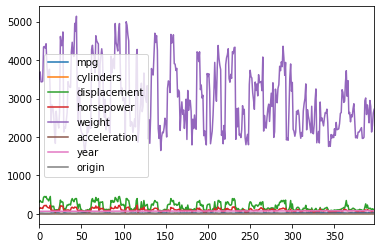In :
data = df.values
data

Out:
array([[18.0, 8, 307.0, ..., 70, 1, 'chevrolet chevelle malibu'],
[15.0, 8, 350.0, ..., 70, 1, 'buick skylark 320'],
[18.0, 8, 318.0, ..., 70, 1, 'plymouth satellite'],
...,
[32.0, 4, 135.0, ..., 82, 1, 'dodge rampage'],
[28.0, 4, 120.0, ..., 82, 1, 'ford ranger'],
[31.0, 4, 119.0, ..., 82, 1, 'chevy s-10']], dtype=object)
In :
data = df.iloc[:, :-1].values
data

Out:
array([[ 18. ,   8. , 307. , ...,  12. ,  70. ,   1. ],
[ 15. ,   8. , 350. , ...,  11.5,  70. ,   1. ],
[ 18. ,   8. , 318. , ...,  11. ,  70. ,   1. ],
...,
[ 32. ,   4. , 135. , ...,  11.6,  82. ,   1. ],
[ 28. ,   4. , 120. , ...,  18.6,  82. ,   1. ],
[ 31. ,   4. , 119. , ...,  19.4,  82. ,   1. ]])
In :
data.dtype

Out:
dtype('float64')
In :
names = names[:-1]

In :
plt.figure(figsize=(10, 10))
nrow, ncol = data.shape
for c in range(ncol):
plt.subplot(3, 3, c+1)
plt.plot(data[:, c])
plt.xlabel('Sample Number')
plt.ylabel(names[c])
# plt.tight_layout()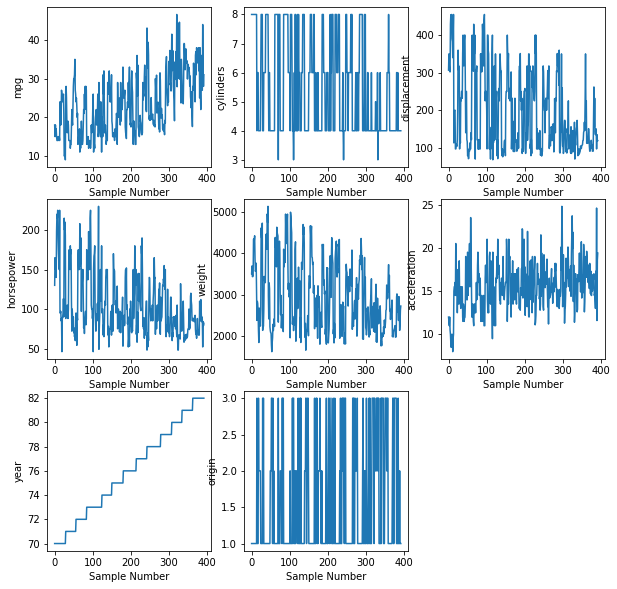What is interesting to you in these graphs?

Let's try to predict miles per gallon, mpg, from the other attributes. To set this up, let's make a 392 x 1 column vector, T, of target values containing all of the mpg values, and a 392 x 7 matrix, X, to hold the inputs to our model.

In :
T = data[:, 0:1]  # data[:,0] results in a one-dimensional matrix, data[:,0:1] preserves its two-dimensional nature.

In :
X = data[:, 1:]

In :
X.shape, T.shape

Out:
((392, 7), (392, 1))
In :
Xnames = names[1:]
Tname = names
Xnames, Tname

Out:
(['cylinders',
'displacement',
'horsepower',
'weight',
'acceleration',
'year',
'origin'],
'mpg')

Now, let's see if a linear model makes some sense by plotting the target values versus each of the input variables.

In :
plt.figure(figsize=(10, 10))
for c in range(X.shape):
plt.subplot(3,3, c+1)
plt.plot(X[:, c], T, 'o', alpha=0.5)
plt.ylabel(Tname)
plt.xlabel(Xnames[c])
plt.tight_layout()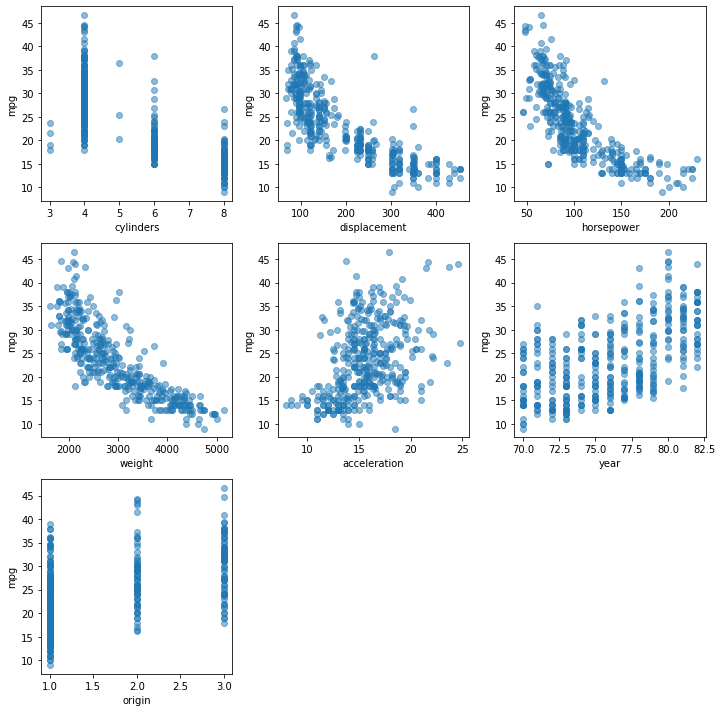What do you think? Are there any linear relationships between the individual input variables and the target variable? Do they make sense, given your knowledge of automobiles?

Now, to build the linear model. First, let's tack on a column of constant 1 values to the left side of X. With this addition, our matrix multiplication takes care of the $w_0$ coefficient as described above. We can use np.insert whose arguments are np.insert(array, where, value, axis), so to add 1's as a column at index 0, we can do this.

In :
X1 = np.insert(X, 0, 1, 1)
X.shape, X1.shape

Out:
((392, 7), (392, 8))
In :
X1[:3, :]

Out:
array([[1.000e+00, 8.000e+00, 3.070e+02, 1.300e+02, 3.504e+03, 1.200e+01,
7.000e+01, 1.000e+00],
[1.000e+00, 8.000e+00, 3.500e+02, 1.650e+02, 3.693e+03, 1.150e+01,
7.000e+01, 1.000e+00],
[1.000e+00, 8.000e+00, 3.180e+02, 1.500e+02, 3.436e+03, 1.100e+01,
7.000e+01, 1.000e+00]])

And, let's add a name to Xnames for the constant 1 column. The $w_0$ weight is often called the "bias" weight, so let's add the name 'bias'.

In :
Xnames.insert(0, 'bias')
Xnames

Out:
['bias',
'cylinders',
'displacement',
'horsepower',
'weight',
'acceleration',
'year',
'origin']

We could try to fit a linear model to all of the data and check to see how accurately we predict mpg for each sample. However, this will give a much too optimistic expectation of how well the model will do on new data.

A much better way to evaluate a model is to remove, or hold out, some of the samples from the data set used to fit the model. Then apply the model to the held out samples and compare the predicted mpg with the actual mpg. Call these held out samples the test set and the other samples used to fit the model the train set.

How should we partition the data into these two disjoint subsets? A common practice is to randomly select 80% of the samples as training samples and use the remaining 20% of the samples as testing samples.

To partition our samples (rows of X and T ) into training and test sets, let's deal with just the row indices. Then we will use the same selected row indices to slice out corresponding rows of X and T.

First let's calculate the number of samples in our training set and testing set.

In :
nrows = X1.shape
nTrain = int(round(nrow * 0.8))
nTest = nrow - nTrain
nTrain, nTest, nTrain + nTest

Out:
(314, 78, 392)

Now we want to randomly select 314 samples (rows) as our training set, and the remaining samples (row) as our testing set. One way to do this is just to randomly permute an array row indices, then select the first 314 for the training set and the remaining 78 as our testing set.

In :
rows = np.arange(nrows)
np.random.shuffle(rows)
rows

Out:
array([163, 285,  52,  55, 131, 203, 316, 224, 107, 235, 356, 151, 358,
21, 215, 332,  77, 282, 263, 325, 385, 196, 105, 280, 353, 381,
184, 250, 118,  99, 387, 341, 312, 305,  11, 207, 275, 123,  33,
117, 303, 269, 343, 347, 378, 335, 383, 227,  67, 264,  13, 389,
69, 314,  24, 334,  44, 374, 317,  22,  62, 216,   4, 294, 338,
212, 132, 140, 357,  43, 180, 238, 345, 194, 360, 101, 278,  46,
369, 254, 301,  81, 247,  70, 344, 186, 307,  82, 158, 286, 248,
322, 137, 111, 244,  23, 142,  93, 179,  29, 121, 336, 166, 292,
223,  14, 164, 368, 225,  73,   0, 249, 252,   8, 259,  94, 354,
153, 170, 315, 167, 352,  15, 306,  27, 125, 232, 346,  86, 141,
329,  28, 297, 217,  90,  36, 309,  89, 130, 313,  12,  83, 120,
210, 154, 268,  31, 340, 320, 330,   5, 289, 211,  61, 218, 331,
276, 364, 149, 157,  25, 241,   9, 246, 270, 150,  80, 376, 324,
37, 124,  79, 205, 119, 229, 209,  39, 226, 365, 139, 321, 199,
87, 367, 160, 102,  85,  20, 366, 200, 257, 176, 128,  38, 361,
115, 304, 189,  98, 161, 308, 116,  17, 391,  40,  75, 147, 359,
204, 319, 372,  48, 291, 265, 198, 233, 208,   1, 349, 339, 190,
258, 187, 214,  84,   6,  78, 159, 256, 273,  10, 106, 103, 144,
34, 277, 311, 302, 221, 143, 375, 281,  54,  47, 213, 148, 197,
267, 327, 373, 193, 222,  60, 188, 326,  95,  72, 202, 390,  91,
113,  41, 271,  51, 110, 253, 318, 138, 295, 234, 351, 136, 298,
63,  26,  74, 172, 183, 255, 300,  97, 236, 377, 260,   3, 192,
35, 370, 177, 191, 162,  30, 108,  57,  64, 122, 323,  56, 112,
18, 342, 185, 206, 243, 181, 355,  32, 380, 348, 371, 171, 240,
53, 228,  68, 293, 168, 174, 290, 104, 175, 129, 178, 284,  19,
382,  88,  50, 310, 287, 239, 169, 155, 145,  92,  65, 388, 274,
16, 114, 242, 288, 201, 362, 152, 195, 262, 384, 296, 219, 237,
251, 133, 279, 261, 266,  42,   2,  58,  45, 231,   7, 328, 283,
220, 146, 100, 272, 182, 127,  71,  49, 299, 337, 135, 230,  66,
245, 363, 165, 109,  59, 379, 126, 333,  96, 156, 350, 386, 134,
76, 173])
In :
trainIndices = rows[:nTrain]
testIndices = rows[nTrain:]
trainIndices, testIndices

Out:
(array([163, 285,  52,  55, 131, 203, 316, 224, 107, 235, 356, 151, 358,
21, 215, 332,  77, 282, 263, 325, 385, 196, 105, 280, 353, 381,
184, 250, 118,  99, 387, 341, 312, 305,  11, 207, 275, 123,  33,
117, 303, 269, 343, 347, 378, 335, 383, 227,  67, 264,  13, 389,
69, 314,  24, 334,  44, 374, 317,  22,  62, 216,   4, 294, 338,
212, 132, 140, 357,  43, 180, 238, 345, 194, 360, 101, 278,  46,
369, 254, 301,  81, 247,  70, 344, 186, 307,  82, 158, 286, 248,
322, 137, 111, 244,  23, 142,  93, 179,  29, 121, 336, 166, 292,
223,  14, 164, 368, 225,  73,   0, 249, 252,   8, 259,  94, 354,
153, 170, 315, 167, 352,  15, 306,  27, 125, 232, 346,  86, 141,
329,  28, 297, 217,  90,  36, 309,  89, 130, 313,  12,  83, 120,
210, 154, 268,  31, 340, 320, 330,   5, 289, 211,  61, 218, 331,
276, 364, 149, 157,  25, 241,   9, 246, 270, 150,  80, 376, 324,
37, 124,  79, 205, 119, 229, 209,  39, 226, 365, 139, 321, 199,
87, 367, 160, 102,  85,  20, 366, 200, 257, 176, 128,  38, 361,
115, 304, 189,  98, 161, 308, 116,  17, 391,  40,  75, 147, 359,
204, 319, 372,  48, 291, 265, 198, 233, 208,   1, 349, 339, 190,
258, 187, 214,  84,   6,  78, 159, 256, 273,  10, 106, 103, 144,
34, 277, 311, 302, 221, 143, 375, 281,  54,  47, 213, 148, 197,
267, 327, 373, 193, 222,  60, 188, 326,  95,  72, 202, 390,  91,
113,  41, 271,  51, 110, 253, 318, 138, 295, 234, 351, 136, 298,
63,  26,  74, 172, 183, 255, 300,  97, 236, 377, 260,   3, 192,
35, 370, 177, 191, 162,  30, 108,  57,  64, 122, 323,  56, 112,
18, 342, 185, 206, 243, 181, 355,  32, 380, 348, 371, 171, 240,
53, 228]),
array([ 68, 293, 168, 174, 290, 104, 175, 129, 178, 284,  19, 382,  88,
50, 310, 287, 239, 169, 155, 145,  92,  65, 388, 274,  16, 114,
242, 288, 201, 362, 152, 195, 262, 384, 296, 219, 237, 251, 133,
279, 261, 266,  42,   2,  58,  45, 231,   7, 328, 283, 220, 146,
100, 272, 182, 127,  71,  49, 299, 337, 135, 230,  66, 245, 363,
165, 109,  59, 379, 126, 333,  96, 156, 350, 386, 134,  76, 173]))

Check that the training and testing sets are disjoint.

In :
np.intersect1d(trainIndices, testIndices)

Out:
array([], dtype=int64)
In :
Xtrain = X1[trainIndices, :]
Ttrain = T[trainIndices, :]
Xtest = X1[testIndices, :]
Ttest = T[testIndices, :]
Xtrain.shape, Ttrain.shape, Xtest.shape, Ttest.shape

Out:
((314, 8), (314, 1), (78, 8), (78, 1))

Now we can use the SGD loop previously shown to find good weights.

First let's add a calculation to track the error. We want to watch how quickly the sum of squared errors, over all samples, is decreasing. More meaningful is the square root of the mean of squared errors (RMSE) so that the units of the error are in the same units of the target variables, rather than the square of them.

Look at the tiny size of the learning_rate. Try editing the value and run the following code cell again.

In :
learning_rate = 0.0000001
n_epochs = 20

n_samples, n_inputs = Xtrain.shape  # number of rows in data equals the number of samples

w = np.zeros((n_inputs, 1))                # initialize the weights to zeros

for epoch in range(n_epochs):             # train for this many epochs, or passes through the data set
sqerror_sum = 0

for n in range(n_samples):
y = Xtrain[n:n + 1, :] @ w      # predicted value, y, for sample n
error = Ttrain[n:n + 1, :] - y
# update weights by fraction of negative derivative of square error with respect to weights
# print(y.shape, error.shape, (X1[n:n+1,:].T * error).shape)
w += learning_rate * Xtrain[n:n + 1, :].T * error
sqerror_sum += error ** 2

rmse = np.sqrt(sqerror_sum / n_samples)
rmse = rmse[0, 0]  # because rmse is 1x1 matrix
print(f'Epoch {epoch + 1} RMSE {rmse:.2f}')

print(w)

Epoch 1 RMSE 19.76
Epoch 2 RMSE 18.41
Epoch 3 RMSE 17.26
Epoch 4 RMSE 16.31
Epoch 5 RMSE 15.53
Epoch 6 RMSE 14.90
Epoch 7 RMSE 14.38
Epoch 8 RMSE 13.96
Epoch 9 RMSE 13.62
Epoch 10 RMSE 13.34
Epoch 11 RMSE 13.11
Epoch 12 RMSE 12.92
Epoch 13 RMSE 12.75
Epoch 14 RMSE 12.61
Epoch 15 RMSE 12.49
Epoch 16 RMSE 12.38
Epoch 17 RMSE 12.28
Epoch 18 RMSE 12.19
Epoch 19 RMSE 12.11
Epoch 20 RMSE 12.03
[[ 0.00132389]
[ 0.00118607]
[-0.12519415]
[-0.00514894]
[ 0.0107926 ]
[ 0.02546657]
[ 0.10785361]
[ 0.00420541]]


Now that we have a linear model, which is simply $w$, let's use it to predict mpg for the first four samples.

In :
predict = Xtrain[:4,:] @ w
predict

Out:
array([[ 9.84355473],
[ 6.90264868],
[18.07197168],
[17.53638638]])

How do these predictions compare with the actual mpg values? We can either make a two column matrix, or use a for loop to print them.

In :
np.hstack(( predict, Ttrain[:4, :]))

Out:
array([[ 9.84355473, 20.        ],
[ 6.90264868, 16.5       ],
[18.07197168, 31.        ],
[17.53638638, 26.        ]])

Go back to the training loop and try different values of learning_rate and n_epochs. Try to find values that result in lowest RMSE.

In :
print('{:^5} {:^5}'.format('P', 'T'))
for (p, t) in zip(predict, Ttrain[0:4, :]):
print('{:5.2f} {:5.2f}'.format(p, t))

  P     T
9.84 20.00
6.90 16.50
18.07 31.00
17.54 26.00


Let's try all of the test data and plot the results.

In :
predict = Xtest @ w
predict.shape, Ttest.shape

Out:
((78, 1), (78, 1))
In :
plt.plot(predict, Ttest, 'o')
plt.xlabel('Predicted MPG')
plt.ylabel('Actual MPG')
# add a 45 degree line
a = max(min(predict), min(Ttest))
b = min(max(predict), max(Ttest))
plt.plot([a, b], [a ,b], 'r', linewidth=3, alpha=0.7);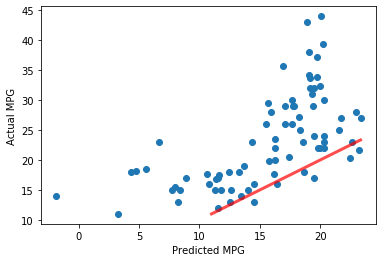Not too shabby! But, how about a numerical measure of accuracy?

Right, just calculate the root-mean-square error (RMSE).

In :
np.sqrt( np.mean( (Xtest @ w - Ttest)**2))

Out:
9.641305787060373

This means we are about 10 mpg off in our predictions, on average.

## Standarizing Variables¶

From trying different values of learning_rate you should have discovered that its value must be very small to prevent the incremental updates to $w$ from resulting in NaN. This is because a larger learning_rate when multiplied by inputs $x$ that are large results in too large a change in $w$ and sends you farther up the opposite side of the bowl that is the quadratic error function we are trying to minimize.

Is there a general way to deal with this problem?

Recall that our variables have widely different ranges, as seen in the plots. Let's make a table of the ranges.

In :
mins = np.min(X1, axis=0)
maxs = np.max(X1, axis=0)
for vi in range(X1.shape):
print(f"{Xnames[vi]:12} {mins[vi]:7.1f}, {maxs[vi]:7.1f}")


bias             1.0,     1.0
cylinders        3.0,     8.0
displacement    68.0,   455.0
horsepower      46.0,   230.0
weight        1613.0,  5140.0
acceleration     8.0,    24.8
year            70.0,    82.0
origin           1.0,     3.0


Since each input value is multiplied by its own weight, the magnitude of the weights are very dependent on the range of its input values. This also means the step size in the gradient descent is very dependent on the range of input values.

To minimize this effect, lets standardize our variables. To do this we will adjust the values of each input variable so that it has a mean of zero and a standard deviation of 1 over all training samples. Of course we do this before tacking on the column of constant 1s, or, equivalently, if the 1s are already there only adjust all columns except the first one.

To do this correctly when partitioning data into training and testing sets, we must always calculate means and standard deviations using only the training set, and use the same means and standard deviations when standardizing the testing set. Remember, you must not use any information about the testing set when building a model. If you do, your test error will be lower than it will be when you truly see new data.

You can do this by storing the means and standard deviations obtained from the training data, and just use them when standardizing the testing data. Don't forget that we don't standardize the column of constant 1s.

In :
Xmeans = np.mean(Xtrain[:, 1:], axis=0)
Xstds = np.std(Xtrain[:, 1:], axis=0)
Xmeans, Xstds

Out:
(array([5.43949045e+00, 1.93479299e+02, 1.04277070e+02, 2.97207325e+03,
1.55191083e+01, 7.59936306e+01, 1.57006369e+00]),
array([1.68105813e+00, 1.04011546e+02, 3.86562054e+01, 8.38055988e+02,
2.70210189e+00, 3.73313075e+00, 8.07919792e-01]))
In :
Xtrain[:, 1:] = (Xtrain[:, 1:] - Xmeans) / Xstds
np.mean(Xtrain, axis=0), np.std(Xtrain, axis=0)

Out:
(array([ 1.00000000e+00, -1.17386638e-16,  2.26287495e-17,  3.18216790e-17,
-1.15972341e-16,  1.41288255e-15, -6.83635738e-16,  3.74788664e-17]),
array([0., 1., 1., 1., 1., 1., 1., 1.]))
In :
Xmeans.shape

Out:
(7,)
In :
Xtest[:, 1:] = (Xtest[:, 1:] - Xmeans) / Xstds

In :
learning_rate = 0.001
n_epochs = 50

n_samples, n_inputs = Xtrain.shape  # number of rows in data equals the number of samples

w = np.zeros((n_inputs, 1))                # initialize the weights to zeros

for epoch in range(n_epochs):             # train for this many epochs, or passes through the data set
sqerror_sum = 0

for n in range(n_samples):
y = Xtrain[n:n + 1, :] @ w      # predicted value, y, for sample n
error = Ttrain[n:n + 1, :] - y
# update weights by fraction of negative derivative of square error with respect to weights
# print(y.shape, error.shape, (X1[n:n+1,:].T * error).shape)
w += learning_rate * Xtrain[n:n + 1, :].T * error
sqerror_sum += error ** 2

rmse = np.sqrt(sqerror_sum / n_samples)
rmse = rmse[0, 0]  # because rmse is 1x1 matrix
print(f'Epoch {epoch + 1} RMSE {rmse:.2f}')

print(w)

Epoch 1 RMSE 21.09
Epoch 2 RMSE 15.35
Epoch 3 RMSE 11.45
Epoch 4 RMSE 8.70
Epoch 5 RMSE 6.80
Epoch 6 RMSE 5.51
Epoch 7 RMSE 4.68
Epoch 8 RMSE 4.17
Epoch 9 RMSE 3.86
Epoch 10 RMSE 3.69
Epoch 11 RMSE 3.59
Epoch 12 RMSE 3.53
Epoch 13 RMSE 3.49
Epoch 14 RMSE 3.47
Epoch 15 RMSE 3.46
Epoch 16 RMSE 3.45
Epoch 17 RMSE 3.44
Epoch 18 RMSE 3.44
Epoch 19 RMSE 3.43
Epoch 20 RMSE 3.43
Epoch 21 RMSE 3.42
Epoch 22 RMSE 3.42
Epoch 23 RMSE 3.42
Epoch 24 RMSE 3.41
Epoch 25 RMSE 3.41
Epoch 26 RMSE 3.41
Epoch 27 RMSE 3.40
Epoch 28 RMSE 3.40
Epoch 29 RMSE 3.40
Epoch 30 RMSE 3.39
Epoch 31 RMSE 3.39
Epoch 32 RMSE 3.39
Epoch 33 RMSE 3.39
Epoch 34 RMSE 3.39
Epoch 35 RMSE 3.38
Epoch 36 RMSE 3.38
Epoch 37 RMSE 3.38
Epoch 38 RMSE 3.38
Epoch 39 RMSE 3.38
Epoch 40 RMSE 3.38
Epoch 41 RMSE 3.37
Epoch 42 RMSE 3.37
Epoch 43 RMSE 3.37
Epoch 44 RMSE 3.37
Epoch 45 RMSE 3.37
Epoch 46 RMSE 3.37
Epoch 47 RMSE 3.37
Epoch 48 RMSE 3.36
Epoch 49 RMSE 3.36
Epoch 50 RMSE 3.36
[[23.53066366]
[-0.43150663]
[ 0.19108164]
[-1.06718848]
[-4.00155849]
[-0.27162365]
[ 2.7718004 ]
[ 0.97878269]]

In :
predict = Xtest @ w
predict.shape, Ttest.shape

Out:
((78, 1), (78, 1))
In :
print('{:^5} {:^5}'.format('P', 'T'))
for (p, t) in zip(predict, Ttest[0:4, :]):
# print(p,t)
print('{:5.2f} {:5.2f}'.format(p, t))

  P     T
11.08 12.00
31.10 35.70
24.61 23.00
21.13 19.00


Let's try all of the test data and plot the results.

In :
predict = Xtest @ w
predict.shape, Ttest.shape

Out:
((78, 1), (78, 1))
In :
plt.plot(predict, Ttest, 'o')
plt.xlabel('Predicted MPG')
plt.ylabel('Actual MPG')
# add a 45 degree line
a = max(min(predict), min(Ttest))
b = min(max(predict), max(Ttest))
plt.plot([a, b], [a ,b], 'r', linewidth=3, alpha=0.7);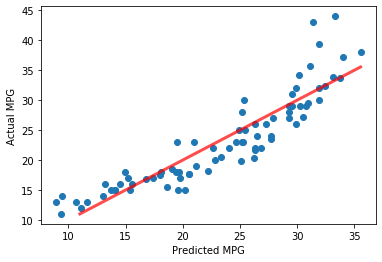That's better. With standardized variables, results are less sensitive to different values of learning_rate.

## Importance of Attributes (Input Variables)¶

Here again are the weights we learned before for predicting mpg. Can we use these to decide which attibutes are most important for predicting mpg? Can we remove some from the model?

A weight magnitude is determined not only by the linear relationship between the corresponding input variable with the target, but also by the variable's range. If we had not standardized the variables, then variables with very small range could result in large magnitude weights, while variables with a large range would result in small magnitude weights. In that case the magnitudes of the weights cannot be used to judge relative importance of variables. However, standardized variables does allow us to compare weight magnitudes to choose important variables.

Let's see what the ranges of our standardized variables are.

In :
mins = np.min(Xtrain, axis=0)
maxs = np.max(Xtrain, axis=0)
for vi in range(Xtrain.shape):
print(f"{Xnames[vi]:12} {mins[vi]:7.1f}, {maxs[vi]:7.1f}")

bias             1.0,     1.0
cylinders       -1.5,     1.5
displacement    -1.2,     2.5
horsepower      -1.5,     3.3
weight          -1.6,     2.6
acceleration    -2.8,     3.4
year            -1.6,     1.6
origin          -0.7,     1.8

In :
for wi, name in zip(w.flat, Xnames):
print('{:8.3f}  {:s}'.format(wi, name))

  23.531  bias
-0.432  cylinders
0.191  displacement
-1.067  horsepower
-4.002  weight
-0.272  acceleration
2.772  year
0.979  origin


Now what do you observe about the relative magnitudes? If you had a ton of input variables, it would be easier to see if we sorted them by their magnitudes.

In :
np.abs(w)

Out:
array([[23.53066366],
[ 0.43150663],
[ 0.19108164],
[ 1.06718848],
[ 4.00155849],
[ 0.27162365],
[ 2.7718004 ],
[ 0.97878269]])
In :
np.argsort(np.abs(w.flat))

Out:
array([2, 5, 1, 7, 3, 6, 4, 0])
In :
np.argsort(np.abs(w.flat))[::-1]  # one way to reverse the order

Out:
array([0, 4, 6, 3, 7, 1, 5, 2])
In :
sortedOrder = np.argsort(np.abs(w.flat))[::-1]
Xnames = np.array(Xnames)
for wi, name in zip(w.flat[sortedOrder], Xnames[sortedOrder]):
print(f'{wi:8.3f}  {name:s}')

  23.531  bias
-4.002  weight
2.772  year
-1.067  horsepower
0.979  origin
-0.432  cylinders
-0.272  acceleration
0.191  displacement


Does this order correspond to what you would expect for predicting mpg? Positive weights indicate that the corresponding variable is positively correlated to mpg, while negative ones indicate a negative correlation.

Which variables might you try removing from the input variables to see if the results are better?

## Multiple Target Components¶

The beauty of matrix equations now shines. Previously $T$ was an $N \times 1$ matrix of target values, one per sample.

Just add additional columns of target values for each target component. So $T$ becomes an $N \times K$ matrix, if there are $K$ output components. Each row is one $K$-dimensional sample.

Let's use this to predict miles per gallon and horsepower.

First, let's assemble the data for predicting MPG and HP.

In :
X = data[:, [1, 2, 4, 5, 6, 7]]
T = data[:, [0, 3]]

Tnames = [names, names]
Xnames = np.array(names)[[1, 2, 4, 5, 6, 7]]

print(X.shape)
print(Xnames)
print(T.shape)
print(Tnames)

(392, 6)
['cylinders' 'displacement' 'weight' 'acceleration' 'year' 'origin']
(392, 2)
['mpg', 'horsepower']

In :
Xtrain = X[trainIndices, :]
Ttrain = T[trainIndices, :]
Xtest = X[testIndices, :]
Ttest = T[testIndices, :]
Xtrain.shape, Ttrain.shape, Xtest.shape, Ttest.shape

Out:
((314, 6), (314, 2), (78, 6), (78, 2))
In :
Xmeans = Xtrain.mean(axis=0)
Xstds = Xtrain.std(axis=0)
Xtrain = (Xtrain - Xmeans) / Xstds
Xtest = (Xtest - Xmeans) / Xstds

Xtrain = np.insert(Xtrain, 0, 1, 1)
Xtest = np.insert(Xtest, 0, 1, 1)

Xnames = np.insert(Xnames, 0, 'bias', 0)
Xnames

Out:
array(['bias', 'cylinders', 'displacement', 'weight', 'acceleration',
'year', 'origin'], dtype='<U12')
In :
Xtrain.shape, Ttrain.shape

Out:
((314, 7), (314, 2))

Now we can train our linear model to predict both mpg and horsepower.

In :
learning_rate = 0.001
n_epochs = 20

n_samples, n_inputs = Xtrain.shape  # number of rows in data equals the number of samples

w = np.zeros((n_inputs, 2))               # initialize the weights to zeros. NOTICE HOW HAS 2 COLUMNS

for epoch in range(n_epochs):             # train for this many epochs, or passes through the data set
sqerror_sum = 0

for n in range(n_samples):
y = Xtrain[n:n + 1, :] @ w      # predicted value, y, for sample n
error = Ttrain[n:n + 1, :] - y
# update weights by fraction of negative derivative of square error with respect to weights
# print(y.shape, error.shape, (X1[n:n+1,:].T * error).shape)
w += learning_rate * Xtrain[n:n + 1, :].T * error
sqerror_sum += error ** 2

rmse = np.sqrt(sqerror_sum / n_samples)
rmse = rmse[0, 0]  # because rmse is 1x1 matrix
print(f'Epoch {epoch + 1} RMSE {rmse:.2f}')

print(w)

Epoch 1 RMSE 21.14
Epoch 2 RMSE 15.38
Epoch 3 RMSE 11.46
Epoch 4 RMSE 8.71
Epoch 5 RMSE 6.81
Epoch 6 RMSE 5.53
Epoch 7 RMSE 4.70
Epoch 8 RMSE 4.19
Epoch 9 RMSE 3.88
Epoch 10 RMSE 3.71
Epoch 11 RMSE 3.61
Epoch 12 RMSE 3.55
Epoch 13 RMSE 3.51
Epoch 14 RMSE 3.49
Epoch 15 RMSE 3.47
Epoch 16 RMSE 3.46
Epoch 17 RMSE 3.46
Epoch 18 RMSE 3.45
Epoch 19 RMSE 3.44
Epoch 20 RMSE 3.44
[[ 2.34891497e+01  1.04031990e+02]
[-8.75167433e-01  4.33058157e+00]
[-9.29674987e-01  1.11367160e+01]
[-3.35792787e+00  1.41801117e+01]
[-1.00840344e-01 -1.19784569e+01]
[ 2.80452188e+00 -3.34941236e+00]
[ 8.41672876e-01  3.59553422e+00]]

In :
Xnames = np.array(Xnames)
for targeti in range(2):
print('\n Weights for {} target\n'.format(Tnames[targeti]))
thisw = w[:, targeti]
sortedOrder = np.argsort(np.abs(thisw))[::-1]
for wi ,name in zip(thisw[sortedOrder], Xnames[sortedOrder]):
print(f'{wi:8.3f}  {name:s}')

 Weights for mpg target

23.489  bias
-3.358  weight
2.805  year
-0.930  displacement
-0.875  cylinders
0.842  origin
-0.101  acceleration

Weights for horsepower target

104.032  bias
14.180  weight
-11.978  acceleration
11.137  displacement
4.331  cylinders
3.596  origin
-3.349  year


Now let's use our single model to predict both mpg and horsepower.

In :
prediction = Xtest @ w
prediction.shape

Out:
(78, 2)
In :
plt.figure(figsize=(10,10))
for p in range(2):
plt.subplot(2, 1, p + 1)
plt.plot(prediction[:, p], Ttest[:, p], 'o')
plt.xlabel("Predicted " + Tnames[p])
plt.ylabel("Actual " + Tnames[p])
a = max(min(prediction[:, p]), min(Ttest[:, p]))
b = min(max(prediction[:, p]), max(Ttest[:, p]))
plt.plot([a, b], [a, b], 'r', linewidth=3)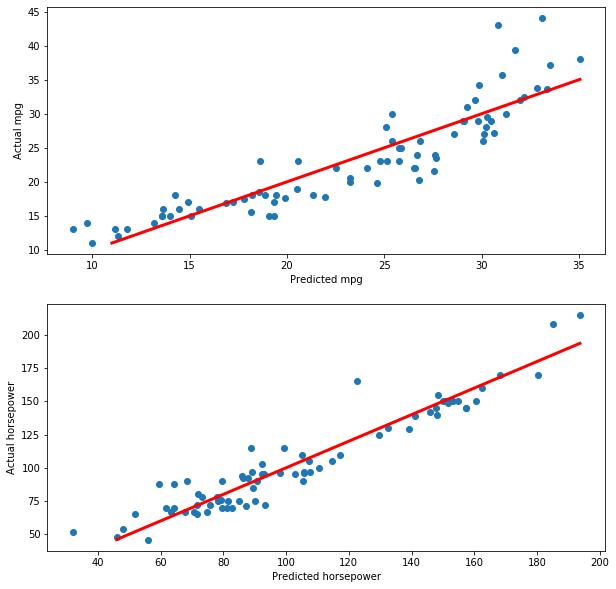How well did we do in terms of RMSE?

In :
rmseTrain = np.sqrt(np.mean((Xtrain @ w - Ttrain)**2, axis=0))
rmseTrain

Out:
array([ 3.42303343, 12.83939267])
In :
rmseTest = np.sqrt(np.mean((Xtest @ w - Ttest)**2, axis=0))
rmseTest

Out:
array([ 3.36866008, 11.46277133])
In :
print('Training RMSE: MPG {:4.2f} HP {:4.2f}'.format(*rmseTrain))   #what is that * doing there??
print(' Testing RMSE: MPG {:4.2f} HP {:4.2f}'.format(*rmseTest))

Training RMSE: MPG 3.42 HP 12.84
Testing RMSE: MPG 3.37 HP 11.46


Looks like we have a bigger error for HP. But maybe this is due to large range of HP.

In :
Ttest.min(0), Ttest.max(0)

Out:
(array([11., 46.]), array([ 44., 215.]))